# KSEEB Solutions for Class 6 Maths Chapter 13 Symmetry Ex 13.3

Students can Download Chapter 13 Symmetry Ex 13.3 Questions and Answers, Notes Pdf, KSEEB Solutions for Class 6 Maths helps you to revise the complete Karnataka State Board Syllabus and score more marks in your examinations.

## Karnataka State Syllabus Class 6 Maths Chapter 13 Symmetry Ex 13.3

Question 1.
Find the number of lines of symmetry in each of the following shapes. How will you check your answers?Solution:a) It can be observed that there are 4 lines of symmetry
b) It can be observed that there is only 1 line of symmetry
c) It can be observed that there are 2 lines of symmetry
d) It can be observed that there are 2 lines of symmetry
e) It can be observed that there is only 1 line of symmetry
f) It can be observed that there are 2 lines of symmetryQuestion 2.
Copy the following drawing on squared paper. Complete each one of them such that the resulting figure has two dotted lines as two lines of symmetry?Solution :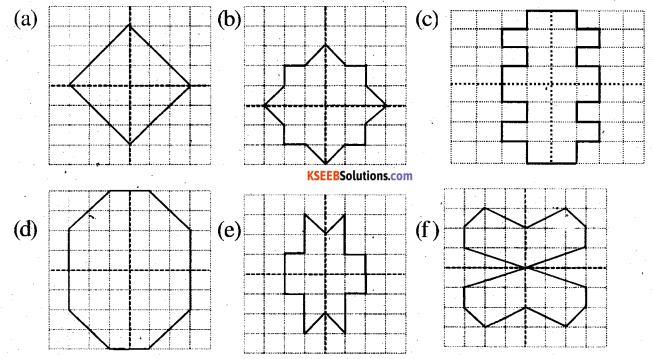Question 3.
In each figure alongside, a letter of the alphabet is shown along with a vertical line. Take the mirror image of the letter in the given line. Find which letters look the same after reflection (i.e. Which letters look the same in the image) and which do not. can you guess why?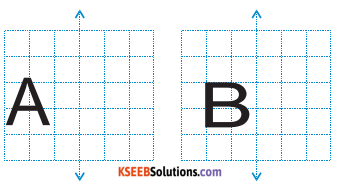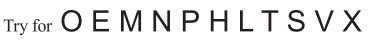Solution: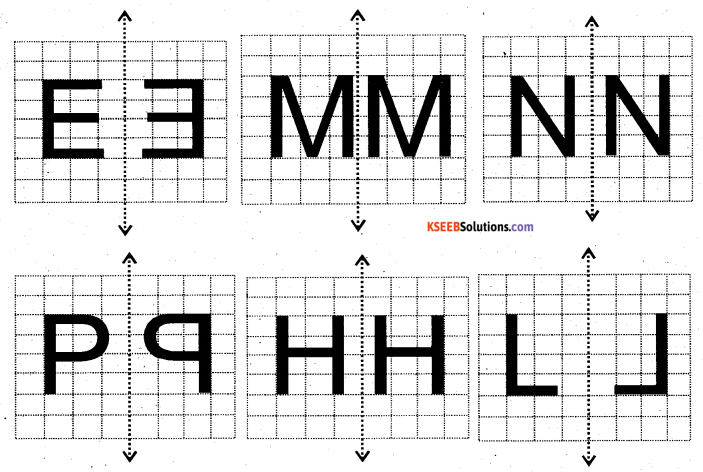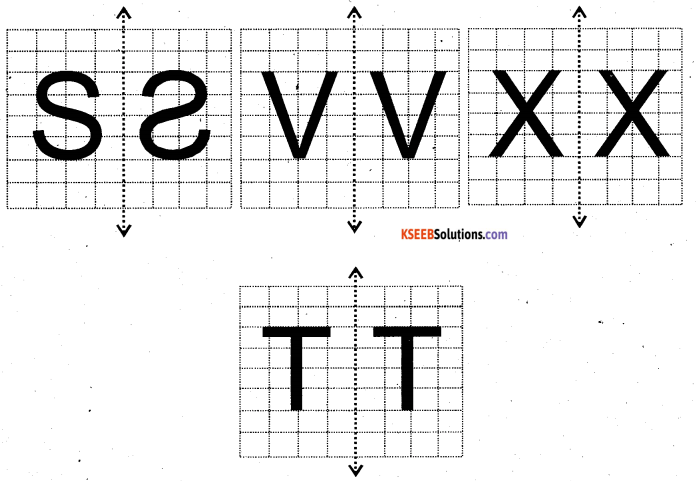error: Content is protected !!